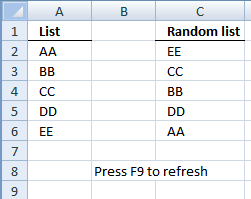Author: Oscar Cronquist Article last updated on April 06, 2011

Overview

The array formula in cell range C2:C6 creates a random list from the values in cell range A2:A6.Array formula in C2:

=INDEX(List, LARGE((ROW(List)-MIN(ROW(List))+1)*(COUNTIF(\$C\$1:C1, List)<>COUNTIF(List, List)), RANDBETWEEN(1, SUM(--(COUNTIF(\$C\$1:C1, List)<>COUNTIF(List, List)))))) + CTRL + SHIFT + ENTER.

How to create an array formula

1. Copy (Ctrl + c) and paste (Ctrl + v) array formula into formula bar.
2. Press and hold Ctrl + Shift.
3. Press Enter once.
4. Release all keys.

Copy cell C2 and paste it down as far as needed.

How this formula works in cell C2

Step 1 - Create an array of row numbers from the named range List

=INDEX(List, LARGE((ROW(List)-MIN(ROW(List))+1)*(COUNTIF(\$C\$1:C1, List)<>COUNTIF(List, List)), RANDBETWEEN(1, SUM(--(COUNTIF(\$C\$1:C1, List)<>COUNTIF(List, List))))))

ROW(reference) returns the rownumber of a reference

(ROW(List)-MIN(ROW(List))+1)

becomes

(ROW(A2:A6)-MIN(ROW(A2:A6))+1)

becomes

{2;3;4;5;6}-MIN(ROW({2;3;4;5;6}))+1

becomes

{2;3;4;5;6}-2+1

becomes

{1;2;3;4;5}

Step 2 - Check value frequency

=INDEX(List, LARGE((ROW(List)-MIN(ROW(List))+1)*(COUNTIF(\$C\$1:C1, List)<>COUNTIF(List, List)), RANDBETWEEN(1, SUM(--(COUNTIF(\$C\$1:C1, List)<>COUNTIF(List, List))))))

COUNTIF(range,criteria) counts the number of cells within a range that meet the given condition

(COUNTIF(\$C\$1:C1, List)<>COUNTIF(List, List))

becomes

(COUNTIF("Random list", {"AA";"DD";"CC";"DD";"EE"})<>COUNTIF(List, List))

becomes

{0;0;0;0;0}<>COUNTIF({"AA";"DD";"CC";"DD";"EE"}, {"AA";"DD";"CC";"DD";"EE"})

becomes

{0;0;0;0;0}<>{1;2;1;2;1}

and returns

{TRUE; TRUE; TRUE; TRUE; TRUE}

Step 3 - Calcultate randbetween arguments

=INDEX(List, LARGE((ROW(List)-MIN(ROW(List))+1)*(COUNTIF(\$C\$1:C1, List)<>COUNTIF(List, List)), RANDBETWEEN(1, SUM(--(COUNTIF(\$C\$1:C1, List)<>COUNTIF(List, List))))))

RANDBETWEEN(bottom, top) returns a random number between the numbers you specify.

RANDBETWEEN(1, SUM(--(COUNTIF(\$C\$1:C1, List)<>COUNTIF(List, List)))))

becomes

RANDBETWEEN(1, SUM(--({0;0;0;0;0}<>{1;2;1;2;1}))))

becomes

RANDBETWEEN(1, SUM(--({TRUE; TRUE; TRUE; TRUE; TRUE}))))

becomes

RANDBETWEEN(1, SUM({1; 1; 1; 1; 1})))

becomes

RANDBETWEEN(1, 5)

and returns a random number between 1 and 5.

Step 4 - Select a random row number

=INDEX(List, LARGE((ROW(List)-MIN(ROW(List))+1)*(COUNTIF(\$C\$1:C1, List)<>COUNTIF(List, List)), RANDBETWEEN(1, SUM(--(COUNTIF(\$C\$1:C1, List)<>COUNTIF(List, List))))))

LARGE(array,k) returns the k-th largest row number in this data set.

becomes

=INDEX(List,LARGE({1;2;3;4;5}, 1))

becomes

=INDEX({"AA";"DD";"CC";"DD";"EE"}, LARGE({1;2;3;4;5}, 1))

and returns EE in cell C2. (random)

How to customize the formula to your excel spreadsheet

Change the named ranges. If your random list starts at cell F2, change cell reference C1:\$C\$1 in the above formula to \$F\$1:F1.

Named ranges

List (A2:A6)
What is named ranges?

Download excel sample file for this tutorial.

sort a list in random order.xls
(Excel 97-2003 Workbook *.xls)

SUMPRODUCT(array1, array2, )
Returns the sum of the products of the corresponding ranges or arrays

COUNTIF(range,criteria)
Counts the number of cells within a range that meet the given condition

LARGE(array,k) returns the k-th largest row number in this data set.

ROW(reference) returns the rownumber of a reference

INDEX(array,row_num,[column_num])
Returns a value or reference of the cell at the intersection of a particular row and column, in a given range

RANDBETWEEN(bottom, top)
Returns a random number between the numbers you specify

ISERROR(value)
Checks whether a value is an error and returns TRUE or FALSE

MATCH(lookup_value;lookup_array; [match_type])
Returns the relative position of an item in an array that matches a specified value

MIN(number1,[number2])
Returns the smallest number in a set of values. Ignores logical values and text